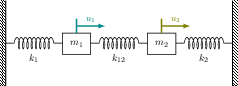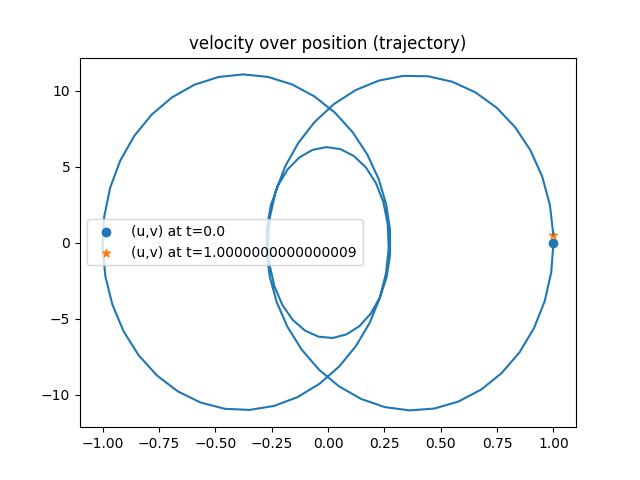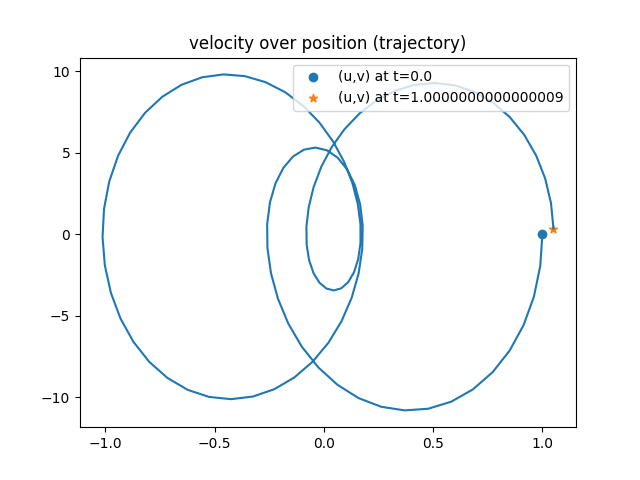We solve an oscillator with two masses in a partitioned fashion. Each mass is solved by an independent ODE.

## Setup

This tutorial solves a simple mass-spring oscillator with two masses and three springs. The system is cut at the middle spring and solved in a partitioned fashion:Note that this case applies a Schwarz-type coupling method and not (like most other tutorials in this repository) a Dirichlet-Neumann coupling. This results in a symmetric setup of the solvers. We will refer to the solver computing the trajectory of $m_1$ as Mass-Left and to the solver computing the trajectory of $m_2$ as Mass-Right. For more information, please refer to .

## Available solvers

This tutorial is only available in Python. You need to have preCICE and the Python bindings installed on your system.

• Python: An example solver using the preCICE Python bindings. This solver also depends on the Python libraries numpy, which you can get from your system package manager or with pip3 install --user <package>.

## Running the Simulation

### Python

Open two separate terminals and start both participants by calling:

cd python
./run.sh -l


and

cd python
./run.sh -r


## Post-processing

Each simulation run creates two files containing position and velocity of the two masses over time. These files are called trajectory-Mass-Left.csv and trajectory-Mass-Right.csv. You can use the script plot-trajectory.py for post-processing. Type python3 plot-trajectory --help to see available options. You can, for example plot the trajectory by running

python3 plot-trajectory.py python/output/trajectory-Mass-Left.csv TRAJECTORY


This allows you to study the effect of different time stepping schemes on energy conservation. Newmark beta conserves energy:Generalized alpha does not conserve energy:For details, refer to .

 V. Schüller, B. Rodenberg, B. Uekermann and H. Bungartz, A Simple Test Case for Convergence Order in Time and Energy Conservation of Black-Box Coupling Schemes, in: WCCM-APCOM2022. URL